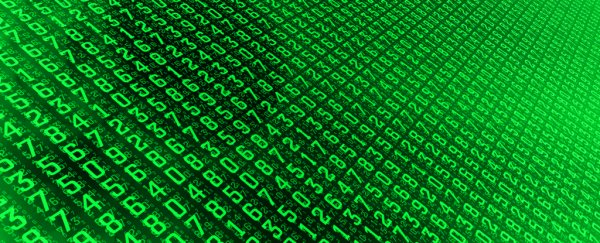(LagartoFilm/iStock)

# Mathematicians Have Discovered an Entirely New Way to Multiply Large Numbers

17 OCTOBER 2019

A pair of mathematicians from Australia and France have devised an alternative way to multiply numbers together, while solving an algorithmic puzzle that has perplexed some of the greatest math minds for almost half a century.

For most of us, the way we multiply relatively small numbers is by remembering our times tables – an incredibly handy aid first pioneered by the Babylonians some 4,000 years ago.

But what if the numbers get bigger? Well, if the figures get unwieldy – and assuming we don't have a calculator or computer, of course – most of us would then turn to long multiplication: another useful trick we learn in school, and a trusty technique for multiplying basically any two numbers together.

There's just one problem with long multiplication. It's slow.

The reason it's slow is because for every single digit in each number in the problem, you need to perform a separate multiplication operation, before adding all the products up.

This might not be a problem for you and me, who probably rarely resort to long multiplication ourselves. But it's a drawback school kids are familiar with, laboriously trudging through their calculations as they learn the magic of multiplication.

More significantly, it's a problem for computers, since their own bottlenecks in performing calculations are imposed by the limits of the abstract mathematics we ourselves can comprehend.

Basically, long multiplication is an algorithm, but it's just not a particularly efficient one, since the process is inevitably painstaking.

As it happens, mathematicians actually have a way of calculating just how painstaking long multiplication is.

As mathematician David Harvey from UNSW in Australia explains in the video below, in a multiplication where both the numbers have 3 digits (n = 3), the number of separate multiplication operations involved is actually 9, which is n2:

The problem with this is that as the numbers get bigger, the amount of work involved scales up too, always being represented by n to the power of 2.

While it's inefficient, the long multiplication algorithm was actually the most advanced multiplication algorithm we had until the 1960s, when Russian mathematician Anatoly Karatsuba discovered that n1.58 was possible.

A decade later, a pair of German mathematicians made another breakthrough: the Schönhage–Strassen algorithm, which conjectured – but never proved – that further refinements were possible, too.

"They predicted that there should exist an algorithm that multiplies n-digit numbers using essentially n * log(n) basic operations," Harvey explains.

"Our paper gives the first known example of an algorithm that achieves this."

According to the researchers, multiplying two numbers together with a billion digits each by the process of long multiplication would take a computer months to calculate.

Using the Schönhage-Strassen algorithm, it would take under 30 seconds, and with their theoretical proof, it would be even quicker – theoretically – and may even represent the fastest multiplication algorithm that's mathematically possible.

"In this sense, our work is expected to be the end of the road for this problem, although we don't know yet how to prove this rigorously," Harvey says.

"People have been hunting for such an algorithm for almost 50 years. It was not a forgone conclusion that someone would eventually be successful."

It's worth noting the new algorithm would only ever be useful for multiplying very big numbers together. How big exactly?

"We have no idea," the researchers explain in an FAQ, although one example they give in the paper equates to 10214857091104455251940635045059417341952, which is a very, very, very big number.

The world's maths community is still absorbing the new findings, which have yet to be peer-reviewed, but are already making waves.

"I was very much astonished that it had been done," theoretical computer scientist Martin Fürer from Penn State University told Science News.

Fürer himself tried to revamp the Schönhage-Strassen algorithm over a decade ago, but eventually discontinued the work, telling Science News, "It seemed quite hopeless to me".

But hope has been restored, provided mathematicians can verify the work.

"If the result is correct, it's a major achievement in computational complexity theory," mathematician and computer scientist Fredrik Johansson from INRIA Bordeaux and Institut de Mathématiques de Bordeaux told New Scientist.

"The new ideas in this work are likely to inspire further research and could lead to practical improvements down the road."

Meanwhile, Harvey and his co-researcher, Joris van der Hoeven from École Polytechnique in France, say their algorithm needs to be optimised, and they're just hoping they haven't stuffed something up in their proof.

"A lot of the work was convincing ourselves that it's actually correct," Harvey told AAP. "I'm still terrified that it might turn out to be wrong."

The pre-print findings are available on the HAL open access archive.

A version of this story was first published in April 2019.